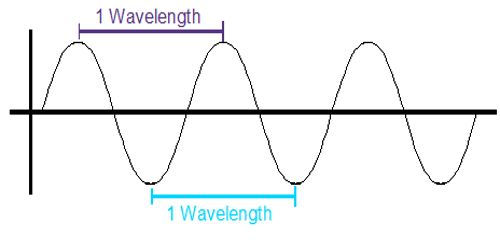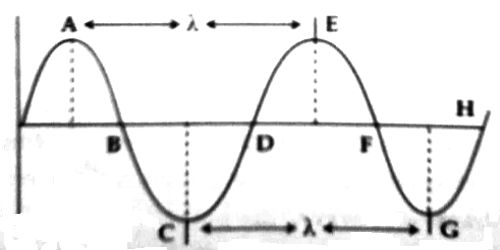# Wavelength

Wavelength: When a wave propagates through a medium, the minimum distance between any two particles of the medium which are in the same phase at any instant is called the wavelength. It is denoted by λ. In Figure, distance between A and E or C and G is the wavelength λ.Wavelength is inversely related to frequency, which refers to the number of wave cycles per second. The higher the frequency of a signal, the shorter the wavelength. A wavelength can be calculated by dividing the velocity of a wave by its frequency.

The vibration caused by a wave, just as a vibration caused by an elastic medium (say, a spring), has an amplitude, a period, and a frequency. So, a wave also is characterized by its frequency, or period, and its amplitude. In addition, a wave is also characterized by its speed of travel.

Another way of taking the wave speed into account is to instead refer to the distance that the wave travels to produce a full cycle of its vibration. This distance is called the wavelength. As the wave moves by, in a time equal to the period one oscillation of the wave occurs and so the wave has moved along a distance equal to the wavelength.